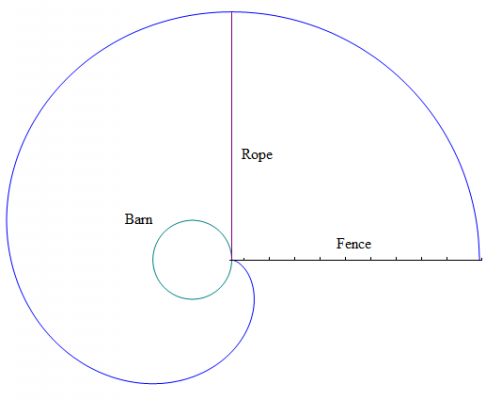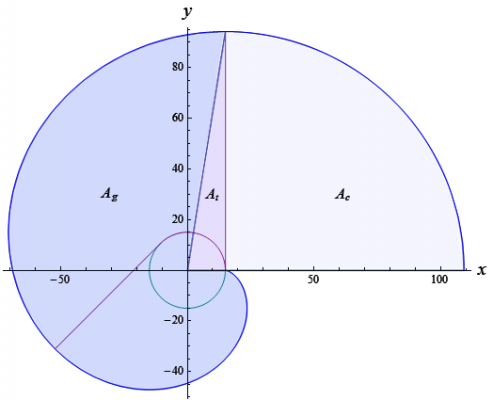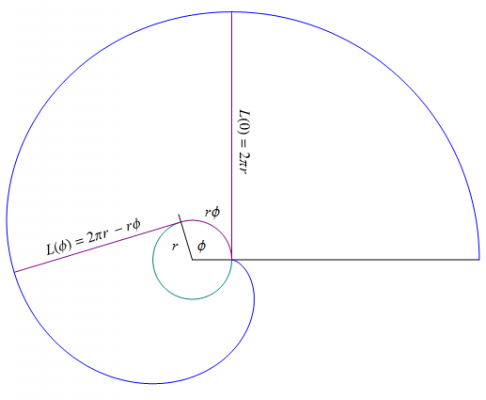## Recommended Posts

This can be a fun problem to solve if you like calculus. There is a cow tethered by a rope to a circular barn on the north side of a fence which extends eastward radially from the barn. The rope and the fence has a length equal to the circumference of the barn. Find the grazing area as a function of the radius of the barn.Edited by Daedalus

##### Share on other sites

I haven't done calculus in a long time, so I hope I don't do anything silly...

Let r = the radius of the barn.

Let R = the length of the rope.

Since the rope wraps around the barn exactly one time,

R = 2*pi*r.

The eastern piece of the area is easy, since it's just a quarter circle with area

(1/4) * pi * R^2

= (1/4) * pi * (2*pi*r)^2

= pi^3 * r^2.

As for the rest, notice that the rope uncurls one point at a time as you move clockwise around the barn. Therefore, integrate along the length of the rope--x ranges from 0 to R.

For a particular x, the distance from the center of the barn is sqrt(x^2 + r^2). Therefore the part of this distance outside the barn is sqrt(x^2 + r^2) - r.

integral{x from 0 to 2*pi*r} (sqrt(x^2 + r^2) - r) dx.

According to my integral tables, this is something awful like

pi * r^2 * [sqrt(pi^2 + 1) - 2] + .5 * r^2 * ln |2*pi + sqrt(4*pi^2 + 1)|

so the total area would be

pi^3 * r^2 + pi * r^2 * [sqrt(pi^2 + 1) - 2] + .5 * r^2 * ln |2*pi + sqrt(4*pi^2 + 1)|

I'd be very surprised if nothing were wrong here though...

##### Share on other sites

I haven't done calculus in a long time, so I hope I don't do anything silly...

Let r = the radius of the barn.

Let R = the length of the rope.

Since the rope wraps around the barn exactly one time,

R = 2*pi*r.

The eastern piece of the area is easy, since it's just a quarter circle with area

(1/4) * pi * R^2

= (1/4) * pi * (2*pi*r)^2

= pi^3 * r^2.

As for the rest, notice that the rope uncurls one point at a time as you move clockwise around the barn. Therefore, integrate along the length of the rope--x ranges from 0 to R.

For a particular x, the distance from the center of the barn is sqrt(x^2 + r^2). Therefore the part of this distance outside the barn is sqrt(x^2 + r^2) - r.

integral{x from 0 to 2*pi*r} (sqrt(x^2 + r^2) - r) dx.

According to my integral tables, this is something awful like

pi * r^2 * [sqrt(pi^2 + 1) - 2] + .5 * r^2 * ln |2*pi + sqrt(4*pi^2 + 1)|

so the total area would be

pi^3 * r^2 + pi * r^2 * [sqrt(pi^2 + 1) - 2] + .5 * r^2 * ln |2*pi + sqrt(4*pi^2 + 1)|

I'd be very surprised if nothing were wrong here though...

Nope that's not the answer : (

##### Share on other sites

From an engineering point of view, the grazing area is about 3 fence-lengths squared, but the rope is just too short.

Also, that fence was put in a really silly place.

##### Share on other sites

The area is r squared, but I haven't specified the units.

##### Share on other sites

The area is 42*C, where C is a constant, which consists of about 1/3rd of everything we know in science, and about 2/3rd of Dark Units.##### Share on other sites

Since no one solved this challenge (or perhaps just didn't care lol), I will go ahead and post the answer.

Define the grazing area in terms of the areas $A_g$ , $A_t$ , $A_c$ , and the area of the barn:

As illustrated in figure 1, the grazing area can be divided into three regions.Figure 1 - A graph of the grazing area, plus the area of the barn, divided into three distinct areas - $A_g$ , $A_t$ , and $A_c$.

$A_g$ is the area defined by integrating the curve in polar coordinates from the angle $\alpha$ to $\beta$:

$A_g = \frac{1}{2} \int_{\alpha}^{\beta} \left | \text{R} \right |^2 \, d\theta \ \ \ \text{where R is a vector valued function.}$

$A_t$ is the area of the triangle excluded by integrating the curve in polar coordinates :

$A_t = \frac{1}{2}\, r \left (2 \pi r\right) = \pi r^2$

$A_c$ is one-fourth the area of a circle with the radius being the full length of the rope:

$A_c = \frac{1}{4}\, \pi \left(2 \pi r\right)^2 = \pi^3 r^2$

The grazing area is the sum of $A_g$ , $A_t$, and $A_c$ minus the area of the barn:

$A® = A_g + A_t + A_c - \pi r^2$

Substituting the values of $A_g$ , $A_t$, and $A_c$ into the equation for the grazing area we get:

$A® = A_g + A_t + A_c - \pi r^2 = \frac{1}{2} \int_{\alpha}^{\beta} \left | \text{R} \right |^2 \, d\theta + \pi^3 r^2$

(Note: $A_t$ and the area of the barn cancel out)

Define the perimeter of $A_g$ as a function of ɸ:

As illustrated in figure 2, the length of the rope wrapped around the barn is $r \phi$, and the length of the rope not touching the barn is $L \left(\phi\right) = 2 \pi r - r \phi = r \left(2 \pi - \phi \right)$:Figure 2 - A graph illustrating the rope as it wraps around the barn.

Since we know that the length of the rope wrapped around the barn is $r \phi$, we can define the position where the rope leaves the surface of the barn as a vector valued function of $\phi$:

$\text{P}\left( \phi \right) = \left \langle \, r \, \text{cos} \, \phi, \ r \, \text{sin} \, \phi \, \right \rangle$

The angle of the rope not touching the barn is equal to $\phi + \pi / 2$. This allows us to define a vector valued function encapsulating the length and angle for this section of the rope as:

$\text{V}\left( \phi \right) = \left \langle \, L\left(\phi \right) \, \text{cos} \left(\phi + \pi / 2\right), \ L\left(\phi \right) \, \text{sin} \left(\phi + \pi / 2\right) \, \right \rangle$

Now that we have defined $\text{P}\left( \phi \right)$ and $\text{V}\left( \phi \right)$ , we can define the perimeter of $A_g$ as follows:

$\text{R}\left( \phi \right) = \text{P}\left( \phi \right) + \text{V}\left( \phi \right)$

Solve for $A_g$ and complete the equation for the grazing area:

Transform $\text{R}\left( \phi \right)$ into polar coordinates so that we can integrate it as a polar function:

$A_g = \frac{1}{2} \int_{\alpha}^{\beta} \left | \text{R} \right |^2 \, d\theta$

The square of the magnitude of $\text{R}\left( \phi \right)$ is the equal to the square of the length of the radius from the center of the barn to the perimeter of $A_g$:

$\left | \text{R} \right |^2 = r^2 \left(1+\left(2 \pi - \phi\right)^2\right)$

The angle of this radius with respect to the $x$ axis is the arctangent of the $y$ component of $\text{R}\left( \phi \right)$ divided by the $x$ component:

$\theta = \text{tan}^{-1} \left( \frac{\left(2 \pi - \phi\right) \text{sin}\left(\phi + \pi / 2\right) + \text{sin} \, \phi}{\left(2 \pi - \phi\right) \text{cos}\left(\phi + \pi / 2\right) + \text{cos} \, \phi} \right)$

Solve for $d\theta$:

$d\theta = \frac{\left(2 \pi - \phi \right)^2}{1+\left(2 \pi - \phi\right)^2} \, d\phi$

Substitute $\left | \text{R} \right |^2$ and $d\theta$ into the equation for $A_g$ (note: since we changed the variable to $\phi$ , we must integrate from $0$ to $2 \pi$:

$A_g = \frac{1}{2} \int_{0}^{2 \pi} r^2 \left(1+\left(2 \pi - \phi\right)^2\right) \frac{\left(2 \pi - \phi \right)^2}{1+\left(2 \pi - \phi \right)^2}\, d\phi = \frac{1}{2} r^2 \int_{0}^{2 \pi} \left(2 \pi - \phi \right)^2 \, d\phi = \frac{4 \pi^3 r^2}{3}$

Substitute $A_g$ into the equation for the grazing area and simplify:

$A® = A_g + \pi^3 r^2 = \frac{4 \pi^3 r^2}{3} + \frac{3 \pi^3 r^2}{3} = \frac{7 \pi^3 r^2}{3}$

Edited by Daedalus

## Create an account

Register a new account